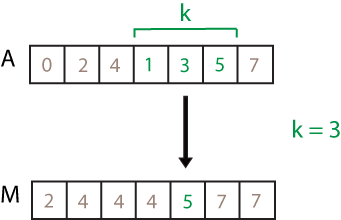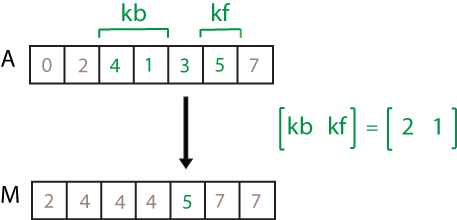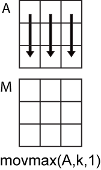movmax

Moving maximum

Description

example

M = movmax(A,k) returns an array of local k-point maximum values, where each maximum is calculated over a sliding window of length k across neighboring elements of A. When k is odd, the window is centered about the element in the current position. When k is even, the window is centered about the current and previous elements. The window size is automatically truncated at the endpoints when there are not enough elements to fill the window. When the window is truncated, the maximum is taken over only the elements that fill the window. M is the same size as A.

• If A is a vector, then movmax operates along the length of the vector.

• If A is a multidimensional array, then movmax operates along the first array dimension whose size does not equal 1.

example

M = movmax(A,[kb kf]) computes the maximum with a window of length kb+kf+1 that includes the element in the current position, kb elements backward, and kf elements forward.

example

M = movmax(___,dim) returns the array of moving maximums along dimension dim for any of the previous syntaxes. For example, if A is a matrix, then movmax(A,k,2) operates along the columns of A, computing the k-element sliding maximum for each row.

example

M = movmax(___,nanflag) specifies whether to include or omit NaN values from the calculation for any of the previous syntaxes. movmax(A,k,'includenan') includes all NaN values in the calculation while movmax(A,k,'omitnan') ignores them and computes the maximum over fewer points.

example

M = movmax(___,Name,Value) specifies additional parameters for the moving maximum using one or more name-value pair arguments. For example, if x is a vector of time values, then movmax(A,k,'SamplePoints',x) computes the moving maximum relative to the times in x.

Examples

collapse all

Compute the three-point centered moving maximum of a row vector. When there are fewer than three elements in the window at the endpoints, take the maximum over the elements that are available.

A = [4 8 6 -1 -2 -3 -1 3 4 5];
M = movmax(A,3)
M = 1×10

8     8     8     6    -1    -1     3     4     5     5

Compute the three-point trailing moving maximum of a row vector. When there are fewer than three elements in the window at the endpoints, movmax takes the maximum over the number of elements that are available.

A = [4 8 6 -1 -2 -3 -1 3 4 5];
M = movmax(A,[2 0])
M = 1×10

4     8     8     8     6    -1    -1     3     4     5

Compute the three-point centered moving maximum for each row of a matrix. The window starts on the first row, slides horizontally to the end of the row, then moves to the second row, and so on. The dimension argument is two, which slides the window across the columns of A.

A = [4 8 6; -1 -2 -3; -1 3 4]
A = 3×3

4     8     6
-1    -2    -3
-1     3     4

M = movmax(A,3,2)
M = 3×3

8     8     8
-1    -1    -2
3     4     4

Compute the three-point centered moving maximum of a row vector containing two NaN elements.

A = [4 8 NaN -1 -2 -3 NaN 3 4 5];
M = movmax(A,3)
M = 1×10

8     8     8    -1    -1    -2     3     4     5     5

Recalculate the maximum, but include the NaN values. When taking the maximum over a group of elements containing at least one NaN value, movmax returns NaN.

M = movmax(A,3,'includenan')
M = 1×10

8   NaN   NaN   NaN    -1   NaN   NaN   NaN     5     5

Compute a 3-hour centered moving maximum of the data in A according to the time vector t.

A = [4 8 6 -1 -2 -3];
k = hours(3);
t = datetime(2016,1,1,0,0,0) + hours(0:5)
t = 1x6 datetime
Columns 1 through 3

01-Jan-2016 00:00:00   01-Jan-2016 01:00:00   01-Jan-2016 02:00:00

Columns 4 through 6

01-Jan-2016 03:00:00   01-Jan-2016 04:00:00   01-Jan-2016 05:00:00

M = movmax(A,k,'SamplePoints',t)
M = 1×6

8     8     8     6    -1    -2

Compute the three-point centered moving maximum of a row vector, but discard any calculation that uses fewer than three points from the output. In other words, return only the maximums computed from a full three-element window, discarding endpoint calculations.

A = [4 8 6 -1 -2 -3 -1 3 4 5];
M = 1×8

8     8     6    -1    -1     3     4     5

Input Arguments

collapse all

Input array, specified as a vector, matrix, or multidimensional array.

Data Types: single | double | int8 | int16 | int32 | int64 | uint8 | uint16 | uint32 | uint64 | logical

Window length, specified as a numeric or duration scalar. When k is a positive integer scalar, the centered maximum includes the element in the current position plus surrounding neighbors. For example, a three-point maximum defined by a window of length three results in the following calculation for a vector A:Data Types: single | double | int8 | int16 | int32 | int64 | uint8 | uint16 | uint32 | uint64 | duration

Directional window length, specified as a numeric or duration row vector containing two elements. When kb and kf are positive integer scalars, the calculation is over kb+kf+1 elements. The calculation includes the element in the current position, kb elements before the current position, and kf elements after the current position. For example, a four-point maximum defined by the directional window [2 1] results in the following calculation for a vector A:Data Types: single | double | int8 | int16 | int32 | int64 | uint8 | uint16 | uint32 | uint64 | duration

Dimension to operate along, specified as a positive integer scalar. If no value is specified, then the default is the first array dimension whose size does not equal 1.

Dimension dim indicates the dimension that movmax operates along, that is, the direction in which the specified window slides.

Consider a two-dimensional input array, A.

• If dim = 1, then movmax(A,k,1) starts with the first column and slides vertically over each row to compute. The maximum is computed over k elements at a time. Then it moves to the second column and repeats the computation. This process continues until all columns are exhausted.• If dim = 2, then movmax(A,k,2) starts with the first row and slides horizontally across each column. The maximum is computed over k elements at a time. Then it moves to the second row and repeats the computation. This process continues until all rows are exhausted.Data Types: double | single | int8 | int16 | int32 | int64 | uint8 | uint16 | uint32 | uint64

NaN condition, specified as one of these values:

• 'omitnan' — Ignore all NaN values in the input. If a window includes only NaN values, then movmax returns NaN.

• 'includenan' — Include NaN values from the input when computing the maximum, resulting in the output NaN.

Name-Value Arguments

Specify optional comma-separated pairs of Name,Value arguments. Name is the argument name and Value is the corresponding value. Name must appear inside quotes. You can specify several name and value pair arguments in any order as Name1,Value1,...,NameN,ValueN.

Example: M = movmax(A,k,'Endpoints','fill')

Method to treat leading and trailing windows, specified as the comma-separated pair consisting of 'Endpoints' and one of the following:

'Endpoints' ValueDescription
'shrink'Shrink the window size near the endpoints of the input to include only existing elements.
'discard'Do not output any maximum values when the window does not completely overlap with existing elements.
'fill'Substitute nonexisting elements with -Inf.
numeric or logical scalarSubstitute nonexisting elements with a specified numeric or logical value.

Data Types: double | single | int8 | int16 | int32 | int64 | uint8 | uint16 | uint32 | uint64 | logical | char | string

Sample points for computing maximums, specified as the comma-separated pair consisting of 'SamplePoints' and a vector. The sample points represent the location of the data in A. Sample points do not need to be uniformly sampled. By default, the sample points vector is [1 2 3 ... ].

Moving windows are defined relative to the sample points, which must be sorted and contain unique elements. For example, if t is a vector of times corresponding to the input data, then movmax(rand(1,10),3,'SamplePoints',t) has a window that represents the time interval between t(i)-1.5 and t(i)+1.5.

When the sample points vector has data type datetime or duration, then the moving window length must have type duration.

If the sample points are nonuniformly spaced and the 'Endpoints' name-value pair is specified, then its value must be 'shrink'.

Data Types: single | double | int8 | int16 | int32 | int64 | uint8 | uint16 | uint32 | uint64 | datetime | duration